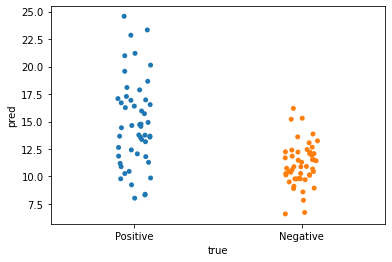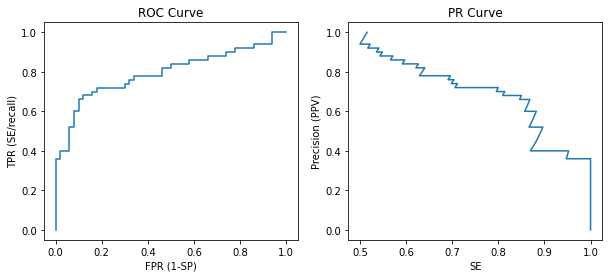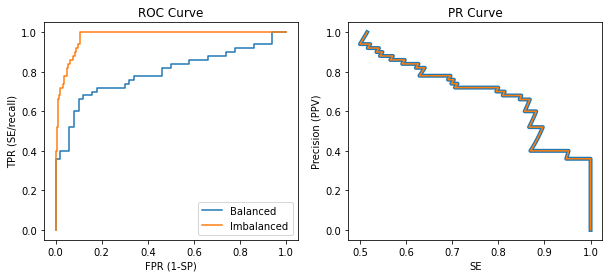### 术语与公式

AUC area under curve 曲面下面积
PRC precision-recall curve 精度-召回率曲线
TP true positive 真阳性预测数量
FP false positive 假阳性预测数量
TN true negative 真阴性预测数量
FN false negative 假阴预测数量
SE sensitivity/recall 敏感度/召回率
SP specificity 特异性
TPR true positive rate 真阳性率，等于 SE
FPR false positive rate 假阳性率，等于 1-SP
PPV positive predictive value 等同于 precision（精度）

$$SE = \frac{TP}{TP+FN}$$

$$SP = \frac{TN}{TN+FP}$$

$$PPV = \frac{TP}{TP+FP}$$

### 基础概念与曲线的绘制预测\真实 患者 健康### ROC 与 PRC 的区别

ROC 可以理解为敏感性随特异性降低而提高的变化趋势。PR 曲线则可以理解为精度随着敏感性升高而变化的趋势。

 曲线 X 轴 Y 轴 指标 公式 指标 公式 PRC 召回率 TP / (TP + FN) 精度 TP / (TP + FP) ROC 特异性 FP / (FP + TN) 敏感性 TP / (TP + FN)

### 数据不平衡下的俩曲线变化